Heat Transfer by Natural Convection - 3

# Heat Transfer by Natural Convection - 3 Notes | Study Heat Transfer - Mechanical Engineering

## Document Description: Heat Transfer by Natural Convection - 3 for Mechanical Engineering 2022 is part of Natural Convection for Heat Transfer preparation. The notes and questions for Heat Transfer by Natural Convection - 3 have been prepared according to the Mechanical Engineering exam syllabus. Information about Heat Transfer by Natural Convection - 3 covers topics like and Heat Transfer by Natural Convection - 3 Example, for Mechanical Engineering 2022 Exam. Find important definitions, questions, notes, meanings, examples, exercises and tests below for Heat Transfer by Natural Convection - 3.

Introduction of Heat Transfer by Natural Convection - 3 in English is available as part of our Heat Transfer for Mechanical Engineering & Heat Transfer by Natural Convection - 3 in Hindi for Heat Transfer course. Download more important topics related with Natural Convection, notes, lectures and mock test series for Mechanical Engineering Exam by signing up for free. Mechanical Engineering: Heat Transfer by Natural Convection - 3 Notes | Study Heat Transfer - Mechanical Engineering
 1 Crore+ students have signed up on EduRev. Have you?

Illustration 5.1

A hot oven is maintained at 180 oC having vertical door 50 cm high is exposed to the atmospheric air at 20oC. Calculate the average heat transfer coefficient at the surface of the door.

The various air properties at the average temperature [(180+20)/2 = 100oC] are,

k = 0.032 W/m oC;      Pr = 0.7;          Kinematic viscosity = 24 x 10-6 m2/s

At Tb = 20oC,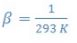Solution 5.1

First we have to find the Grashof number,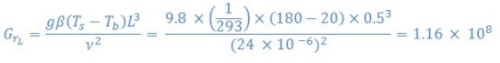With the help of Gr and Pr, we can estimate the Ra number,

Ra = GrPr = 1.16 X 108 X 0.7 = 8.12 X 107

As Ra < 109, the eq.5.7 can be used,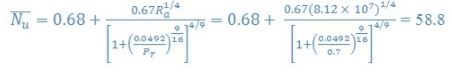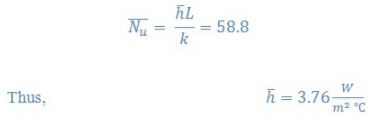5.3 Combined natural and forced convection

As we know that there is hardly any situation in which only natural or forced convective heat transfer occurs. Generally, in all the processes natural and forced convection heat transfer occur but depending upon the contribution made, the process may be approximated as either natural or forced convection problem.  However, certain situation needs to be addressed as combined natural and forced convection problem. The following is a thumb rule to determine the individual situations.

1. Forced convection region i.e. negligible natural convection contribution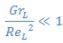2. Natural convection region i.e. negligible forced convection contribution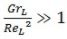3. Mixed convection i.e., significant contribution by both natural and forced convections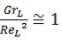In this situation, the following equation may be used,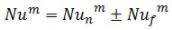where, Nu is the Nusselt number due to mixed convection, Nun is the Nusselt number due to natural convection, and Nuf is the Nusselt number due to forced convection. The value of m is generally taken as 3, whereas positive and negative signs can be used for the convection in the same and opposite directions, respectively.

Illustration 5.2

In the oven door described in illustration 5.1 is subjected to an upward flow of air (that is forced convection). What would be the minimum free stream velocity for which natural convection may be neglected?

Solution 5.2.

Section 5.3 above shows that for the following condition the effect of natural convection may be neglected,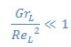The value of Gr number calculated in the previous illustration was 1.16 X 108

Thus,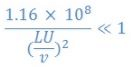U >> 0.24 m/s

Therefore, the bulk velocity of the air should be far greater that 0.24 m/s.

The document Heat Transfer by Natural Convection - 3 Notes | Study Heat Transfer - Mechanical Engineering is a part of the Mechanical Engineering Course Heat Transfer.
All you need of Mechanical Engineering at this link: Mechanical Engineering

## Heat Transfer

58 videos|70 docs|85 tests
 Use Code STAYHOME200 and get INR 200 additional OFF

## Heat Transfer

58 videos|70 docs|85 tests

Track your progress, build streaks, highlight & save important lessons and more!

,

,

,

,

,

,

,

,

,

,

,

,

,

,

,

,

,

,

,

,

,

;